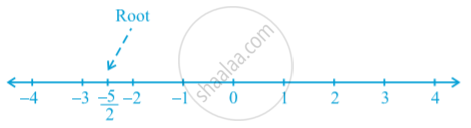# Linear Equation in Two Variables

#### notes

Consider the following equation: 2x + 5 = 0
the root of the equation, is  -5/2. This can be represented on the number line as shown below:While solving an equation, you must always keep the following points in mind:
The solution of a linear equation is not affected when:
(i) the same number is added to (or subtracted from) both the sides of the equation.
(ii) you multiply or divide both the sides of the equation by the same non-zero number.
So, any equation which can be put in the form ax + by + c = 0, where a, b and c are real numbers, and a and b are not both zero, is called a linear equation in two variables.

#### notes

Linear equation is a algebric equation having degree as 1, we can say 2x+5=0 is a linear equation, as the degree of the variable x is 1. But in linear equation in two variable have two variables. Eg. 2x+5y-1=0 is in the form of linear equation with two variables x and y. ax+by+c=0 is a standard form of linear equation in two variables, here a is the coefficient of x, b is the coefficient of y and c is a constant term, a,b and c are real numbers, also a and b are not equal to zero.
In this chapter we have to learn about the Pair of Linear Equations in Two Variables. Generally Pair of Linear Equations in Two Variables are written as a_1x+b_1y+c_1=0 and a_2x+b_2y+c_2=0. Example 2x+9y+12=0 and 6x+1y+8=0
To learn how to solve Pair of Linear Equations in Two Variables there are two methods one is Graphical and other is Algebraic. We will study this in the following concepts.

If you would like to contribute notes or other learning material, please submit them using the button below.

### Shaalaa.com

Pair of Linear Equation in two variable part 1 (Introduction) [00:12:13]
S
0%